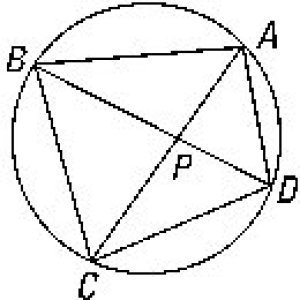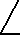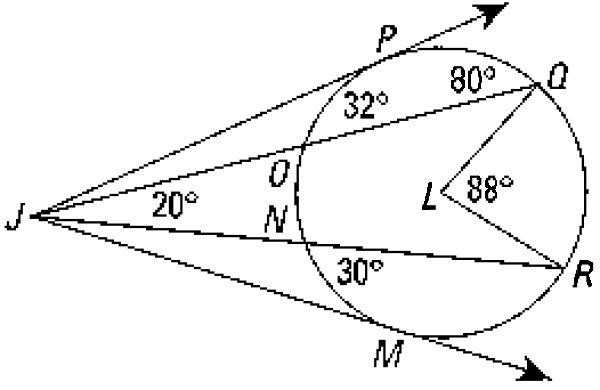Name:                                              Score:

## Chapter 14 Homework (Review), Due Monday, May 22, 2006

 This chapter review is best done without referring to the text or notes and by yourself. However, you may use such references at your own risk of not mastering the material. Each question is worth 10 points. Do both odd and even numbered questions! DO NOT TURN THIS SHEET IN. For full credit, be sure to SHOW COMPLETE SOLUTIONS NOT JUST ANSWERS.

1. A regular hexagon is inscribed in a circle of radius 9. What is the area between the circle and the hexagon? Do and leave answer in radical form!

2. A regular pentagon is inscribed in a circle. If the radius of the circle is 12", what is the length of a side of the pentagon?

3. What is the area exterior to the pentagon but inside the circle as described above?

4. A circle has radius 40mm. Find the length of a chord of an 80° arc.5. If the mABD = 70° and mBAD=80°, find the measure of major arc(BAD) and mBCD.

6. Find mBAD and mADB when arc(AB)= 100° and arc(AD)=50°.

7. If mCPD=40° and the arc(CD)=37°, what is the measure of the arc(AB)?

8. A circle and a square have perimeter of 16". Calculate the radius of the circle and the length of a side of the square. Calculate the areas of both (exactly). How much bigger, in percent, is the circle than the square?9. Assume segment PT is tangent to circle O at T, length PT is 10, and length PO is 20. What is the area of the circle?, the distance from P to N?

10. Assume the earth is a perfect sphere (O) with radius 3960 miles. From the top (P) of the TV 9 & 10 transmission tower south of Cadillac, 2999 feet above "sea level" (N), how far can you see (T)? (cf14.R#44)

11. Assume you have a camera with a lens having a 62° field of vision, and you want to fit an entire monument of width 45 yards into your picture. How far from the monument must you stand? (cf14.3#4)12. Using circle L as marked, find mPJQ and mPJM.